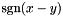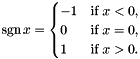# cu/int.h: Integer Functions [Data Types]

## Defines

#define cu_uint16_ntoh   cu_uint16_hton
#define cu_uint32_ntoh   cu_uint32_hton

## Functions

int cu_int_min (int x, int y)
int cu_int_max (int x, int y)
unsigned int cu_uint_min (unsigned int x, unsigned int y)
unsigned int cu_uint_max (unsigned int x, unsigned int y)
long cu_long_min (long x, long y)
long cu_long_max (long x, long y)
unsigned long cu_ulong_min (unsigned long x, unsigned long y)
unsigned long cu_ulong_max (unsigned long x, unsigned long y)
intptr_t cu_intptr_min (intptr_t x, intptr_t y)
intptr_t cu_intptr_max (intptr_t x, intptr_t y)
uintptr_t cu_uintptr_min (uintptr_t x, uintptr_t y)
uintptr_t cu_uintptr_max (uintptr_t x, uintptr_t y)
int cu_int_abs (int x)
long cu_long_abs (long x)
int cu_int_sgn (int x)
int cu_long_sgn (int x)
int cu_int_cmp (int x, int y)
int cu_long_cmp (long x, long y)
unsigned int cu_uint_ceil_div (unsigned int x, unsigned int y)
unsigned long cu_ulong_ceil_div (unsigned long x, unsigned long y)
uint_fast8_t cu_uint8_dcover (uint_fast8_t x) CU_ATTR_PURE
uint_fast16_t cu_uint16_dcover (uint_fast16_t x) CU_ATTR_PURE
uint_fast32_t cu_uint32_dcover (uint_fast32_t x) CU_ATTR_PURE
uint_fast64_t cu_uint64_dcover (uint_fast64_t x) CU_ATTR_PURE
unsigned int cu_uint_dcover (unsigned int x)
unsigned long cu_ulong_dcover (unsigned long x)
uintptr_t cu_uintptr_dcover (uintptr_t x)
unsigned int cu_uint_ucover (unsigned int x)
unsigned long cu_ulong_ucover (unsigned long x)
unsigned int cu_uint_lzeros (unsigned int x)
unsigned long cu_ulong_lzeros (unsigned long x)
unsigned int cu_uint_uzeros (unsigned int x)
unsigned long cu_ulong_uzeros (unsigned long x)
uint_fast8_t cu_uint8_exp2_ceil_log2 (uint_fast8_t x)
uint_fast16_t cu_uint16_exp2_ceil_log2 (uint_fast16_t x)
uint_fast32_t cu_uint32_exp2_ceil_log2 (uint_fast32_t x)
uint_fast64_t cu_uint64_exp2_ceil_log2 (uint_fast64_t x)
unsigned int cu_uint_exp2_ceil_log2 (unsigned int x)
unsigned long cu_ulong_exp2_ceil_log2 (unsigned long x)
unsigned int cu_uint8_bit_count (uint_fast8_t x) CU_ATTR_PURE
unsigned int cu_uint16_bit_count (uint_fast16_t x) CU_ATTR_PURE
unsigned int cu_uint32_bit_count (uint_fast32_t x) CU_ATTR_PURE
unsigned int cu_uint64_bit_count (uint_fast64_t x) CU_ATTR_PURE
unsigned int cu_uint_bit_count (unsigned int x)
unsigned long cu_ulong_bit_count (unsigned long x)
unsigned int cu_uint8_floor_log2 (uint_fast8_t x) CU_ATTR_PURE
unsigned int cu_uint16_floor_log2 (uint_fast16_t x) CU_ATTR_PURE
unsigned int cu_uint32_floor_log2 (uint_fast32_t x) CU_ATTR_PURE
unsigned int cu_uint64_floor_log2 (uint_fast64_t x) CU_ATTR_PURE
unsigned int cu_uint_floor_log2 (unsigned int x)
unsigned int cu_ulong_floor_log2 (unsigned long x)
unsigned int cu_uint8_ceil_log2 (uint_fast8_t x) CU_ATTR_PURE
unsigned int cu_uint16_ceil_log2 (uint_fast16_t x) CU_ATTR_PURE
unsigned int cu_uint32_ceil_log2 (uint_fast32_t x) CU_ATTR_PURE
unsigned int cu_uint64_ceil_log2 (uint_fast64_t x) CU_ATTR_PURE
unsigned int cu_uint_ceil_log2 (unsigned int x)
unsigned int cu_ulong_ceil_log2 (unsigned long x)
unsigned int cu_uint8_log2_lowbit (uint_fast8_t x)
unsigned int cu_uint16_log2_lowbit (uint_fast16_t x)
unsigned int cu_uint32_log2_lowbit (uint_fast32_t x)
unsigned int cu_uint64_log2_lowbit (uint_fast64_t x)
unsigned int cu_uint_log2_lowbit (unsigned int x)
unsigned int cu_ulong_log2_lowbit (unsigned long x)
unsigned int cu_uintptr_log2_lowbit (uintptr_t x)
uint16_t cu_uint16_hton (uint16_t i)
uint32_t cu_uint32_hton (uint32_t i)

## Function Documentation

 int cu_int_abs ( int x )

The absolute value af x.

 int cu_int_cmp ( int x, int y )

Returns, which may be different from cu_long_sgn(x - y) due to overflow.

 int cu_int_max ( int x, int y )

The larger or x and y.

 int cu_int_min ( int x, int y )

The smaller of x and y.

 int cu_int_sgn ( int x )

Returns the signum function of x,intptr_t cu_intptr_max ( intptr_t x, intptr_t y )

The larger or x and y.

 intptr_t cu_intptr_min ( intptr_t x, intptr_t y )

The smaller of x and y.

 long cu_long_abs ( long x )

The absolute value af x.

 int cu_long_cmp ( long x, long y )

Returns, which may be different from cu_long_sgn(x - y) due to overflow.

 long cu_long_max ( long x, long y )

The larger or x and y.

 long cu_long_min ( long x, long y )

The smaller of x and y.

 int cu_long_sgn ( int x )

Returns the signum function of x, cf cu_int_sgn.

 unsigned int cu_uint16_bit_count ( uint_fast16_t x )

Returns the number of high bits in x.

 unsigned int cu_uint16_ceil_log2 ( uint_fast16_t x )

Returns ⌈log2 x⌉.

 uint_fast16_t cu_uint16_dcover ( uint_fast16_t x )

Returns a bitmask from the uppermost non-zero bit in x and downwards.

 uint_fast16_t cu_uint16_exp2_ceil_log2 ( uint_fast16_t x )

Returns 2⌈log2 x.

 unsigned int cu_uint16_floor_log2 ( uint_fast16_t x )

Returns the exponent of the uppermost non-zero bit in x, that is ⌊log2 x⌋.

 unsigned int cu_uint16_log2_lowbit ( uint_fast16_t x )

Returns the exponent of the lowermost non-zero bit in x.

 unsigned int cu_uint32_bit_count ( uint_fast32_t x )

Returns the number of high bits in x.

 unsigned int cu_uint32_ceil_log2 ( uint_fast32_t x )

Returns ⌈log2 x⌉.

 uint_fast32_t cu_uint32_dcover ( uint_fast32_t x )

Returns a bitmask from the uppermost non-zero bit in x and downwards.

 uint_fast32_t cu_uint32_exp2_ceil_log2 ( uint_fast32_t x )

Returns 2⌈log2 x.

 unsigned int cu_uint32_floor_log2 ( uint_fast32_t x )

Returns the exponent of the uppermost non-zero bit in x, that is ⌊log2 x⌋.

 unsigned int cu_uint32_log2_lowbit ( uint_fast32_t x )

Returns the exponent of the lowermost non-zero bit in x.

 unsigned int cu_uint64_bit_count ( uint_fast64_t x )

Returns the number of high bits in x.

 unsigned int cu_uint64_ceil_log2 ( uint_fast64_t x )

Returns ⌈log2 x⌉.

 uint_fast64_t cu_uint64_dcover ( uint_fast64_t x )

Returns a bitmask from the uppermost non-zero bit in x and downwards.

 uint_fast64_t cu_uint64_exp2_ceil_log2 ( uint_fast64_t x )

Returns 2⌈log2 x.

 unsigned int cu_uint64_floor_log2 ( uint_fast64_t x )

Returns the exponent of the uppermost non-zero bit in x, that is ⌊log2 x⌋.

 unsigned int cu_uint64_log2_lowbit ( uint_fast64_t x )

Returns the exponent of the lowermost non-zero bit in x.

 unsigned int cu_uint8_bit_count ( uint_fast8_t x )

Returns the number of high bits in x.

 unsigned int cu_uint8_ceil_log2 ( uint_fast8_t x )

Returns ⌈log2 x⌉.

 uint_fast8_t cu_uint8_dcover ( uint_fast8_t x )

Returns a bitmask from the uppermost non-zero bit in x and downwards.

 uint_fast8_t cu_uint8_exp2_ceil_log2 ( uint_fast8_t x )

Returns 2⌈log2 x.

 unsigned int cu_uint8_floor_log2 ( uint_fast8_t x )

Returns the exponent of the uppermost non-zero bit in x, that is ⌊log2 x⌋.

 unsigned int cu_uint8_log2_lowbit ( uint_fast8_t x )

Returns the exponent of the lowermost non-zero bit in x.

 unsigned int cu_uint_bit_count ( unsigned int x )

Returns the number of high bits in x.

 unsigned int cu_uint_ceil_div ( unsigned int x, unsigned int y )

Returns ⌈x/y⌉.

 unsigned int cu_uint_ceil_log2 ( unsigned int x )

Returns ⌈log2 x⌉.

 unsigned int cu_uint_dcover ( unsigned int x )

Returns a bitmask from the uppermost non-zero bit in x and downwards.

 unsigned int cu_uint_exp2_ceil_log2 ( unsigned int x )

Returns 2⌈log2 x.

 unsigned int cu_uint_floor_log2 ( unsigned int x )

Returns the exponent of the uppermost non-zero bit in x, that is ⌊log2 x⌋.

 unsigned int cu_uint_log2_lowbit ( unsigned int x )

Returns the exponent of the lowermost non-zero bit in x.

 unsigned int cu_uint_lzeros ( unsigned int x )

A bitmask which covers the lowermost continuous zeros of x.

 unsigned int cu_uint_max ( unsigned int x, unsigned int y )

The larger or x and y.

 unsigned int cu_uint_min ( unsigned int x, unsigned int y )

The smaller of x and y.

 unsigned int cu_uint_ucover ( unsigned int x )

Returns a bitmask from the lowermost non-zero bit in x and upwards.

 unsigned int cu_uint_uzeros ( unsigned int x )

A bitmask which covers the uppermost continuous zeroes of x.

 uintptr_t cu_uintptr_dcover ( uintptr_t x )

Returns a bitmask from the uppermost non-zero bit in x and downwards.

 unsigned int cu_uintptr_log2_lowbit ( uintptr_t x )

Returns the exponent of the lowermost non-zero bit in x.

 uintptr_t cu_uintptr_max ( uintptr_t x, uintptr_t y )

The larger or x and y.

 uintptr_t cu_uintptr_min ( uintptr_t x, uintptr_t y )

The smaller of x and y.

 unsigned long cu_ulong_bit_count ( unsigned long x )

Returns the number of high bits in x.

 unsigned long cu_ulong_ceil_div ( unsigned long x, unsigned long y )

Returns ⌈x/y⌉.

 unsigned int cu_ulong_ceil_log2 ( unsigned long x )

Returns ⌈log2 x⌉.

 unsigned long cu_ulong_dcover ( unsigned long x )

Returns a bitmask from the uppermost non-zero bit in x and downwards.

 unsigned long cu_ulong_exp2_ceil_log2 ( unsigned long x )

Returns 2⌈log2 x.

 unsigned int cu_ulong_floor_log2 ( unsigned long x )

Returns the exponent of the uppermost non-zero bit in x, that is ⌊log2 x⌋.

 unsigned int cu_ulong_log2_lowbit ( unsigned long x )

Returns the exponent of the lowermost non-zero bit in x.

 unsigned long cu_ulong_lzeros ( unsigned long x )

A bitmask which covers the lowermost continuous zeros of x.

 unsigned long cu_ulong_max ( unsigned long x, unsigned long y )

The larger or x and y.

 unsigned long cu_ulong_min ( unsigned long x, unsigned long y )

The smaller of x and y.

 unsigned long cu_ulong_ucover ( unsigned long x )

Returns a bitmask from the lowermost non-zero bit in x and upwards.

 unsigned long cu_ulong_uzeros ( unsigned long x )

A bitmask which covers the uppermost continuous zeroes of x.

Generated 2009-11-23 for culibs-0.25 using Doxygen. Maintained by Petter Urkedal.